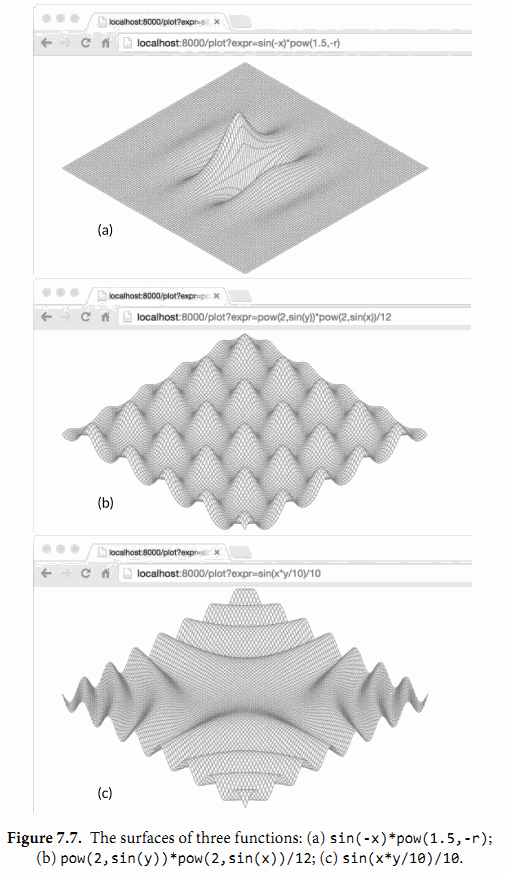## 7.9. 示例: 表达式求值

// An Expr is an arithmetic expression.
type Expr interface{}


sqrt(A / pi)
pow(x, 3) + pow(y, 3)
(F - 32) * 5 / 9


gopl.io/ch7/eval

// A Var identifies a variable, e.g., x.
type Var string

// A literal is a numeric constant, e.g., 3.141.
type literal float64

// A unary represents a unary operator expression, e.g., -x.
type unary struct {
op rune // one of '+', '-'
x  Expr
}

// A binary represents a binary operator expression, e.g., x+y.
type binary struct {
op   rune // one of '+', '-', '*', '/'
x, y Expr
}

// A call represents a function call expression, e.g., sin(x).
type call struct {
fn   string // one of "pow", "sin", "sqrt"
args []Expr
}


type Env map[Var]float64


type Expr interface {
// Eval returns the value of this Expr in the environment env.
Eval(env Env) float64
}


func (v Var) Eval(env Env) float64 {
return env[v]
}

func (l literal) Eval(_ Env) float64 {
return float64(l)
}


unary和binary的Eval方法会递归的计算它的运算对象，然后将运算符op作用到它们上。我们不将被零或无穷数除作为一个错误，因为它们都会产生一个固定的结果——无限。最后，call的这个方法会计算对于pow，sin，或者sqrt函数的参数值，然后调用对应在math包中的函数。

func (u unary) Eval(env Env) float64 {
switch u.op {
case '+':
return +u.x.Eval(env)
case '-':
return -u.x.Eval(env)
}
panic(fmt.Sprintf("unsupported unary operator: %q", u.op))
}

func (b binary) Eval(env Env) float64 {
switch b.op {
case '+':
return b.x.Eval(env) + b.y.Eval(env)
case '-':
return b.x.Eval(env) - b.y.Eval(env)
case '*':
return b.x.Eval(env) * b.y.Eval(env)
case '/':
return b.x.Eval(env) / b.y.Eval(env)
}
panic(fmt.Sprintf("unsupported binary operator: %q", b.op))
}

func (c call) Eval(env Env) float64 {
switch c.fn {
case "pow":
return math.Pow(c.args.Eval(env), c.args.Eval(env))
case "sin":
return math.Sin(c.args.Eval(env))
case "sqrt":
return math.Sqrt(c.args.Eval(env))
}
panic(fmt.Sprintf("unsupported function call: %s", c.fn))
}


func TestEval(t *testing.T) {
tests := []struct {
expr string
env  Env
want string
}{
{"sqrt(A / pi)", Env{"A": 87616, "pi": math.Pi}, "167"},
{"pow(x, 3) + pow(y, 3)", Env{"x": 12, "y": 1}, "1729"},
{"pow(x, 3) + pow(y, 3)", Env{"x": 9, "y": 10}, "1729"},
{"5 / 9 * (F - 32)", Env{"F": -40}, "-40"},
{"5 / 9 * (F - 32)", Env{"F": 32}, "0"},
{"5 / 9 * (F - 32)", Env{"F": 212}, "100"},
}
var prevExpr string
for _, test := range tests {
// Print expr only when it changes.
if test.expr != prevExpr {
fmt.Printf("\n%s\n", test.expr)
prevExpr = test.expr
}
expr, err := Parse(test.expr)
if err != nil {
t.Error(err) // parse error
continue
}
got := fmt.Sprintf("%.6g", expr.Eval(test.env))
fmt.Printf("\t%v => %s\n", test.env, got)
if got != test.want {
t.Errorf("%s.Eval() in %v = %q, want %q\n",
test.expr, test.env, got, test.want)
}
}
}


go test(§11.1) 命令会运行一个包的测试用例：

\$ go test -v gopl.io/ch7/eval


sqrt(A / pi)
map[A:87616 pi:3.141592653589793] => 167

pow(x, 3) + pow(y, 3)
map[x:12 y:1] => 1729
map[x:9 y:10] => 1729

5 / 9 * (F - 32)
map[F:-40] => -40
map[F:32] => 0
map[F:212] => 100


type Expr interface {
Eval(env Env) float64
// Check reports errors in this Expr and adds its Vars to the set.
Check(vars map[Var]bool) error
}


func (v Var) Check(vars map[Var]bool) error {
vars[v] = true
return nil
}

func (literal) Check(vars map[Var]bool) error {
return nil
}

func (u unary) Check(vars map[Var]bool) error {
if !strings.ContainsRune("+-", u.op) {
return fmt.Errorf("unexpected unary op %q", u.op)
}
return u.x.Check(vars)
}

func (b binary) Check(vars map[Var]bool) error {
if !strings.ContainsRune("+-*/", b.op) {
return fmt.Errorf("unexpected binary op %q", b.op)
}
if err := b.x.Check(vars); err != nil {
return err
}
return b.y.Check(vars)
}

func (c call) Check(vars map[Var]bool) error {
arity, ok := numParams[c.fn]
if !ok {
return fmt.Errorf("unknown function %q", c.fn)
}
if len(c.args) != arity {
return fmt.Errorf("call to %s has %d args, want %d",
c.fn, len(c.args), arity)
}
for _, arg := range c.args {
if err := arg.Check(vars); err != nil {
return err
}
}
return nil
}

var numParams = map[string]int{"pow": 2, "sin": 1, "sqrt": 1}


x % 2               unexpected '%'
math.Pi             unexpected '.'
!true               unexpected '!'
"hello"             unexpected '"'

log(10)             unknown function "log"
sqrt(1, 2)          call to sqrt has 2 args, want 1


Check方法的参数是一个Var类型的集合，这个集合聚集从表达式中找到的变量名。为了保证成功的计算，这些变量中的每一个都必须出现在环境变量中。从逻辑上讲，这个集合就是调用Check方法返回的结果，但是因为这个方法是递归调用的，所以对于Check方法，填充结果到一个作为参数传入的集合中会更加的方便。调用方在初始调用时必须提供一个空的集合。

gopl.io/ch7/surface

import "gopl.io/ch7/eval"

func parseAndCheck(s string) (eval.Expr, error) {
if s == "" {
return nil, fmt.Errorf("empty expression")
}
expr, err := eval.Parse(s)
if err != nil {
return nil, err
}
vars := make(map[eval.Var]bool)
if err := expr.Check(vars); err != nil {
return nil, err
}
for v := range vars {
if v != "x" && v != "y" && v != "r" {
return nil, fmt.Errorf("undefined variable: %s", v)
}
}
return expr, nil
}


func plot(w http.ResponseWriter, r *http.Request) {
r.ParseForm()
expr, err := parseAndCheck(r.Form.Get("expr"))
if err != nil {
http.Error(w, "bad expr: "+err.Error(), http.StatusBadRequest)
return
}
w.Header().Set("Content-Type", "image/svg+xml")
surface(w, func(x, y float64) float64 {
r := math.Hypot(x, y) // distance from (0,0)
return expr.Eval(eval.Env{"x": x, "y": y, "r": r})
})
}